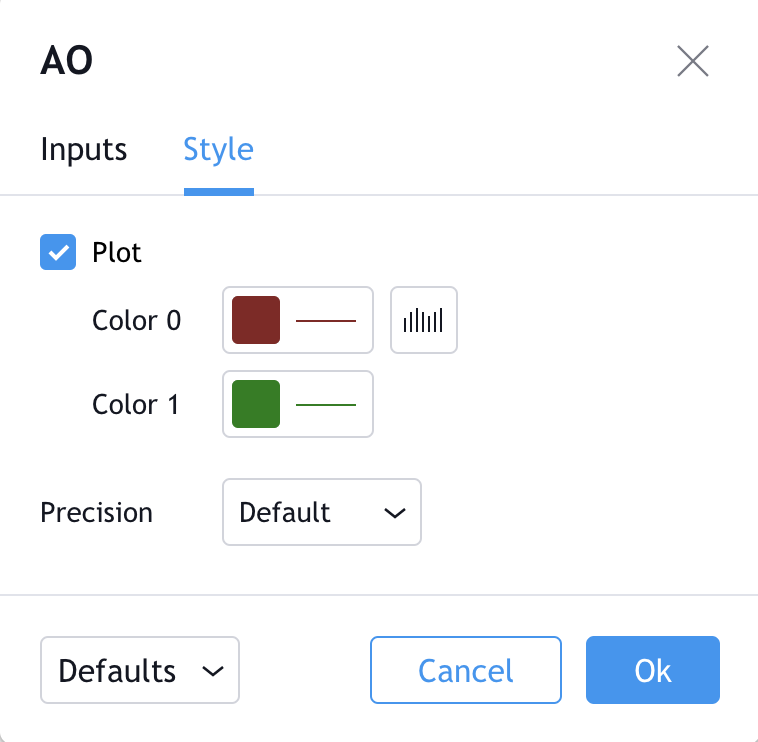# Awesome Oscillator (AO)

#### Definition

The Awesome Oscillator is an indicator used to measure market momentum. AO calculates the difference of a 34 Period and 5 Period Simple Moving Averages. The Simple Moving Averages that are used are not calculated using closing price but rather each bar's midpoints. AO is generally used to affirm trends or to anticipate possible reversals.

#### History

The Awesome Oscillator was created by Bill Williams.

#### Calculation

```lengthAO1=input(5, minval=1) //5 periods
lengthAO2=input(34, minval=1) //34 periods
AO = sma((high+low)/2, lengthAO1) - sma((high+low)/2, lengthAO2)```

#### The basics

Because of its nature as an oscillator, The Awesome Oscillator is designed to have values that fluctuate above and below a Zero Line. The generated values are plotted as a histogram of red and green bars. A bar is green when its value is higher than the previous bar. A red bar indicates that a bar is lower than the previous bar. When AO's values are above the Zero Line, this indicates that the short term period is trending higher than the long term period. When AO's values are below the Zero Line, the short term period is trending lower than the Longer term period. This information can be used for a variety of signals.

#### What to look for

##### Zero Line Cross

The most straightforward, basic signal generated by the Awesome Indicator is the Zero Line Cross. This is simply when the AO value crosses above or below the Zero Line. This indicates a change in momentum.

When AO crosses above the Zero Line, short term momentum is now rising faster than the long term momentum. This can present a bullish buying opportunity.

When AO crosses below the Zero Line, short term momentum is now falling faster then the long term momentum. This can present a bearish selling opportunity.

##### Twin Peaks

Twin Peaks is a method which considers the differences between two peaks on the same side of the Zero Line.

A Bullish Twin Peaks setup occurs when there are two peaks below the Zero Line. The second peak is higher than the first peak and followed by a green bar. Also very importantly, the trough between the two peaks, must remain below the Zero Line the entire time.

A Bearish Twin Peaks setup occurs when there are two beaks above the Zero Line. The second peak is lower than the first peak and followed by a red bar. The trough between both peaks, must remain above the Zero Line for the duration of the setup.

##### Saucer

A Saucer AO Setup looks for more rapid changes in momentum. The Saucer method looks for changes in three consecutive bars, all on the same side of the Zero Line.

A Bullish Saucer setup occurs when the AO is above the Zero Line. It entails two consecutive red bars (with the second bar being lower than the first bar) being followed by a green Bar.

A Bearish Saucer setup occurs when the AO is below the Zero Line. It entails two consecutive green bars (with the second bar being higher than the first bar) being followed by a red bar.

#### Summary

All in all, The Awesome Oscillator can be a fairly valuable tool. momentum is one of those aspects of the market that is crucial to understanding price movements, yet it is so hard to get a solid grip on. AO (momentum) can be used in some instances to generate quality signals but much like with any signal generating indicator, it should be used with caution. Truly understanding the setups and avoiding false signals is something that the best traders learn through experience over time. That being said, the Awesome Indicator produces quality information and may be a valuable technical analysis tool for many analysts or traders.

#### Style##### Growing

Can change the Growing (Up) Bar's color and thickness as well as the indicator's visual type (Histogram is the default). Can also toggle the visibility of a price line showing the current value of the Awesome Oscillator.

##### Falling

Can change the Falling (Down) Bar's color and thickness.

##### Precision

Sets the number of decimal places to be left on the indicator's value before rounding up. The higher this number, the more decimal points will be on the indicator's value.